# Difference AP 4

Calculate the difference of the AP if a1 = 0.5, a2 + a3 = -1.1

Result

d =  -0.7

#### Solution:Leave us a comment of example and its solution (i.e. if it is still somewhat unclear...):

Showing 0 comments:Be the first to comment!#### To solve this example are needed these knowledge from mathematics:

Do you have a linear equation or system of equations and looking for its solution? Or do you have quadratic equation?

## Next similar examples:

1. Difference AP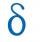Calculate the difference of arithmetic progression if the sum of its first 19 members Sn = 8075 and the first member is a1 = 20
2. Determine AP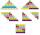Determine the difference of the arithmetic progression if a3 = 7, and a4 + a5 = 71
3. Fifth memberDetermine the fifth member of the arithmetic progression, if the sum of the second and fifth members equal to 73, and difference d = 7.
4. AS sequenceIn an arithmetic sequence is given the difference d = -3 and a71 = 455. a) Determine the value of a62 b) Determine the sum of 71 members.
5. The sum 2The sum of five consecutive even integers is 150. Find the largest of the five integers. A.28 B.30 C.34 D.54 Show your solution and explain your answer.
6. Tubes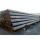Iron tubes in the warehouse are stored in layers so that each tube top layer fit into the gaps of the lower layer. How many layers are needed to deposit 100 tubes if top layer has 9 tubes? How many tubes are in bottom layer of tubes?
7. Cinema 4In cinema are 1656 seats and in the last row are 105 seats , in each next row 3 seats less. How many are the total rows in cinema?
8. Crystal water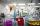The chemist wanted to check the content of water of crystallization of chromic potassium alum K2SO4 * Cr2 (SO4) 3 * 24 H2O, which was a long time in the laboratory. From 96.8 g of K2SO4 * Cr2 (SO4) 3 * 24 H2O prepared 979 cm3 solution of base. S
9. Evaluation of expressionsIf a2-3a+1=0, find (i)a2+1/a2 (ii) a3+1/a3
10. Square root 2If the square root of 3m2 +22 and -x = 0, and x=7, what is m?
11. Parametric equationFind the parametric equation of a line with y-intercept (0,-4) and a slope of -2.
12. Third memberDetermine the third member of the AP if a4=93, d=7.5.
13. SequenceBetween numbers 1 and 53 insert n members of the arithmetic sequence that its sum is 702.
14. Sequence 3Write the first 5 members of an arithmetic sequence: a4=-35, a11=-105.
15. SeatsSeats in the sport hall are organized so that each subsequent row has five more seats. First has 10 seats. How many seats are: a) in the eighth row b) in the eighteenth row
16. AS - sequence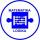What are the first ten members of the sequence if a11=22, d=2.
17. Theorem proveWe want to prove the sentense: If the natural number n is divisible by six, then n is divisible by three. From what assumption we started?